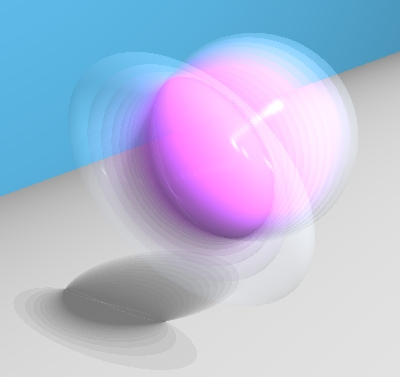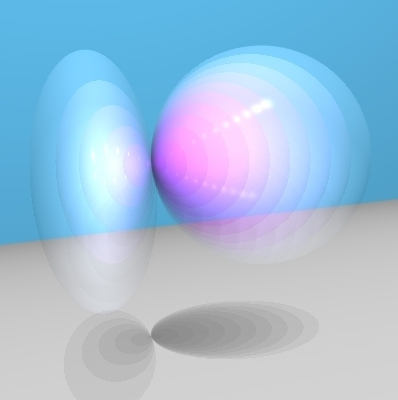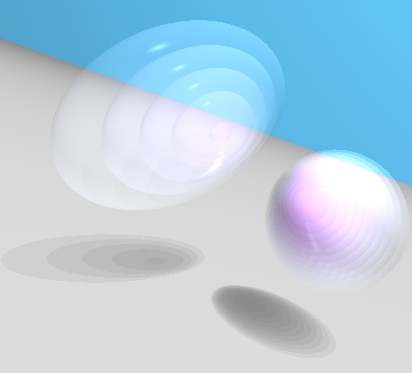Linear Systems of Ellipsoids

A "quadric surface" in three-dimensional space is the solution set of an equation of the form:
Ax2 + By2 + Cz2 + 2Fyz + 2Gxz + 2Hxy + 2Px + 2Qy + 2Rz + D = 0
for constant coefficients A, B, C, F, G, H, P, Q, R, D. There are different types of quadric surfaces in real space, including cylinders, cones, spheres, ellipsoids, paraboloids, hyperboloids, and a few more. If you know the coefficients, you can find the geometric type of the quadric surface, by using the table from a Geometry Center web page. We can abbreviate the equation as E1(x,y,z) = 0, and multiplying all the coefficients of E1 by the same nonzero constant, c, gives
(c . E1)(x,y,z) = cAx2 + cBy2 + cCz2 + 2cFyz + 2cGxz + 2cHxy + 2cPx + 2cQy + 2cRz + cD.
Then E1(x,y,z) = 0 if and only if (c . E1)(x,y,z) = 0, so E1 = 0 and c . E1 = 0 have the same solution set, and they define the same quadric surface.

Linear systems.
A "linear system of quadrics" is the set of linear combinations of linearly independent polynomials E1 and E2:
t1 . E1 + t2 . E2.
Plugging specific numbers into these coefficients, (t1,t2), gives a polynomial in (x,y,z), so each ordered pair (t1,t2) corresponds to a quadric surface (t1 . E1 + t2 . E2)(x,y,z) = 0. Multiplying both t1 and t2 by the same nonzero constant c results in the same surface:
((c . t1) . E1 + (c . t2) . E2)(x,y,z)=c . (t1 . E1 + t2 . E2)(x,y,z) = 0.
So, the non-zero pairs (t1,t2) could be considered as homogeneous coordinates for a projective line. (See my Steiner surfaces page for some details on homogeneous coordinates.) An "affine" linear system is where t1 and t2 are linear functions of just one parameter t (the two functions should be linearly independent). Two such choices are:
• E1 + t . E2. At t = 0, this defines the quadric E1 = 0. For nonzero t, E1 + t . E2 and (1/t) . E1 + E2 define the same quadric, which approaches E2 = 0 as t approaches infinity.
• (1 - t) . E1 + t . E2. This gives the quadric E1 = 0 at t = 0, and the quadric E2 = 0 at t=1.
Geometrically, the idea is that two quadrics, E1 = 0 and E2 = 0, are given, and the linear system is a set of quadrics "connecting" these two, deforming one into the other as t varies.

Intersections.
A point (x,y,z) is on both quadrics if E1(x,y,z) = 0 and E2(x,y,z) = 0. Any point on this intersection will also be a point on every quadric in the linear system: for any t1, t2,
(t1 . E1 + t2 . E2)(x,y,z) = t1 . 0 + t2 . 0 = 0.
In fact, if any two quadrics in a linear system meet at a point, then that point is on every member of the linear system. If two quadrics in a linear system are disjoint, then every pair of quadrics in the linear system will also be disjoint.

Ellipsoids.
In the following examples, both of the polynomials E1 and E2 define ellipsoids. I have also chosen the coefficients so that the values of E1(x,y,z) and E2(x,y,z) are negative for (x,y,z) in the interior. The surfaces will demonstrate the (1 - t) . E1 + t . E2 system, for 0 <= t <= 1.These ellipsoids meet along a space curve.The ellipsoids in this system meet at one point, where they have a common tangent plane. For one value of t between 0 and 1, the quadric is just one point: the equation ((1 - t) . E1 + t . E2)(x,y,z) = 0 has exactly one solution.The ellipsoids E1 and E2 are disjoint, with disjoint interiors. Some of the quadrics in this linear systems are ellipsoids, but there are two values of t between 0 and 1, where the quadric is just one point. For t between these two values, the quadric is "empty" because there are no real solutions of ((1 - t) . E1 + t . E2)(x,y,z) = 0. These empty quadrics could be called "imaginary ellipsoids," since their equations have some complex solutions. In the complex projective coordinate system, any quadric equation (with coefficients A, B, C not all zero) has infinitely many solutions, and any two quadric surfaces have a non-empty intersection.

I've given a couple undergraduate math club talks related to this topic:

Back to the graphics gallery.